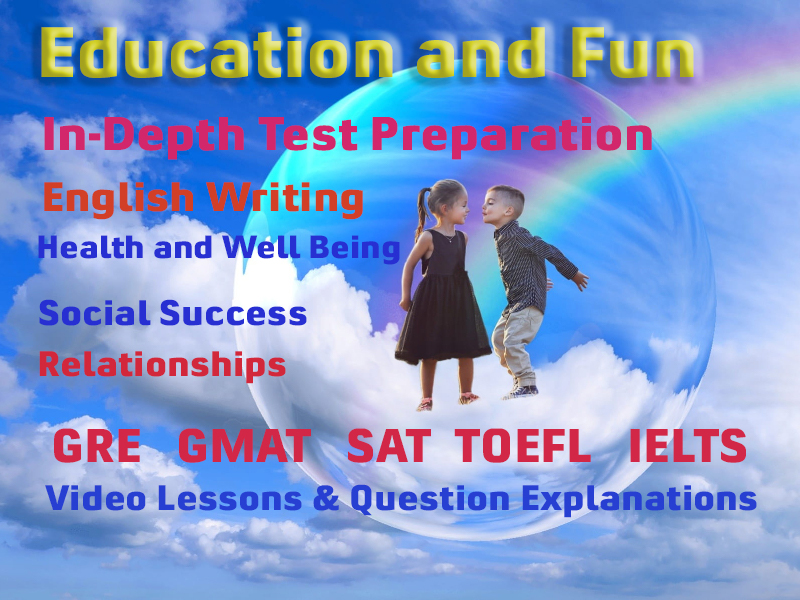# Applied Arithmetic

In any math problem on any aptitude test the question is actually covered in a mask of situation. You interpret the it and formulized to an arithmetic expression. Think; if you understand the situation and formulate the expression correctly but make mistakes in calculating the value. You answer will be wrong. So the arithmetic calculations are the most important to answer correctly.

In this module, each topic and method of solution is presented and explained with emphasis on quick solutions.

### What is the Question?

Read the problem carefully. Make sure you answer the actual question. Many time a student gives an answer in miles when the question asks for miles per hour. Nobody sees your calculations but you. Only the correct answer counts. Be careful.

Most often students having passed 12 grade with mathematics try to involve the formulas they have learnt in college. For instance; to find the part of circumference of a circle don't use S = rθ.

### Video Lessons and 10 Fully Explained Grand Tests

Large number of solved practice MCQ with explanations. Video Lessons and 10 Fully explained Grand/Full Tests.

### More Educational and Fun StuffMore In-depth knowledge about what you need. Detailed about test preparation, English Writing, TOEFL, and IELTS.